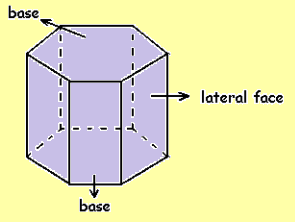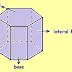Home » Geometry » Prism Formulas for Competitive Exams

# Prism Formulas for Competitive Exams

## Prism Formulas for Competitive Exams

### What is Prism?

A polyhedron with two polygonal
bases parallel to each other is a prism. In optics, the prism is the
transparent optical element with flat polished surfaces that refract light.
Lateral faces join the two polygonal bases, and these lateral faces are mostly
rectangles. It may be a parallelogram in some cases.There are two formulas of the prism.

1. The
surface area of a prism = (2×BaseArea) +Lateral Surface Area

2. The
volume of a prism = Base Area× Height

### Types of Prism

There are different types of prisms. Some of them are:

1. Rectangular Prism: In a Rectangular Prism, 2 rectangular bases are
parallel to each other and 4 rectangular faces.

The base area of a Rectangular prism = bl

The
surface area of a Rectangular prism = 2(bl+lh+hb)

The
volume of a Rectangular prism = lbh

Where b=Base
length, l=base width, h=height

2. Triangular
Prism:
In a Triangular Prism, there are 2 parallel triangular surfaces, 2
rectangular surfaces that are inclined to each other and 1 rectangular
base.

The base area of a Triangular prism = (½)ab

The surface area of a Triangular prism = ab+3bh

The volume of a Triangular prism = (½)abh

Where, a = Apothem length, b=base length, h=height

3. Pentagonal
Prism:
In a Pentagonal Prism, 2 pentagonal surfaces are parallel to each
other and 5 rectangular surfaces that are inclined to each other.

The base area of Pentagonal prism = (5/2)ab

The surface area of a Pentagonal prism = 5ab+5bh

The volume of a Pentagonal prism = (5/2)abh

Where, a
= Apothem length, b=base length, h=height

4. Hexagonal
Prism:
In a Hexagonal Prism, there are 2 hexagonal surfaces parallel to
each other and 6 rectangular surfaces that are inclined to each other.

The base area of hexagonal prism = 3ab

The surface area of a Hexagonal prism = 6ab+6bh

The volume of a Hexagonal prism = 3abh

Where, a
= Apothem length, b=base length, h=height

Prism Formulas for Competitive ExamsReviewed by SSC NOTES
on

November 13, 2021

Rating: 5

“);